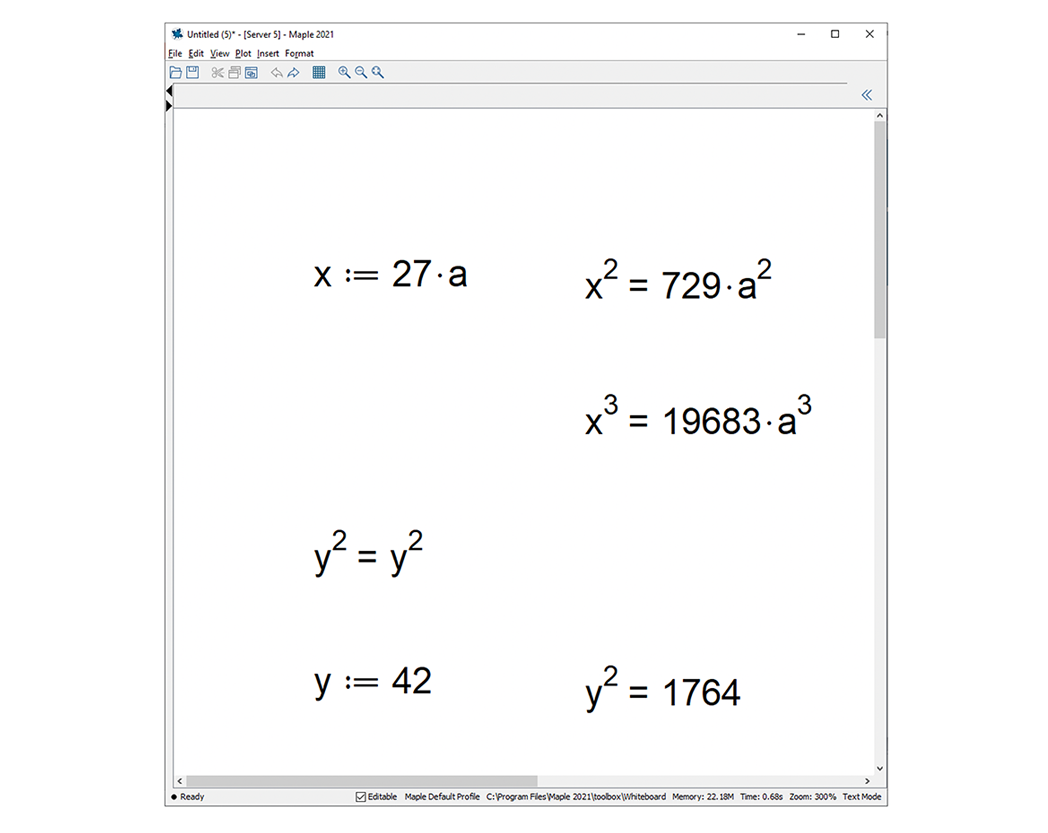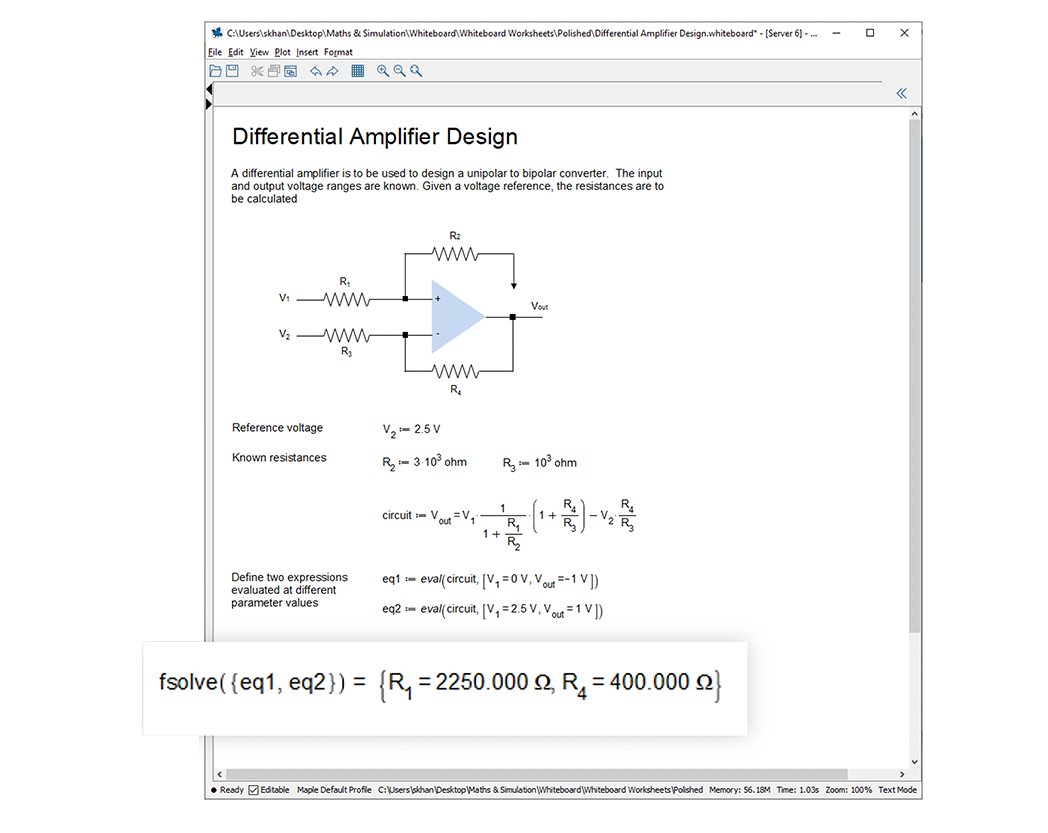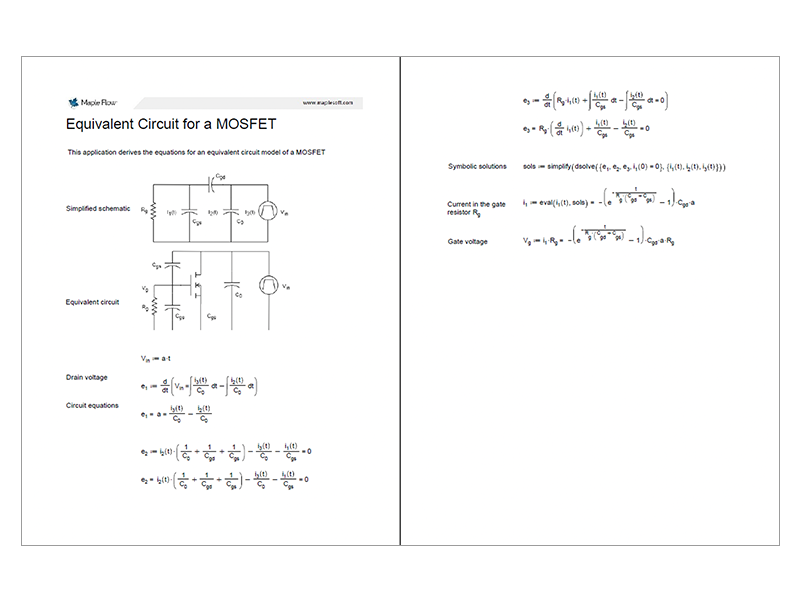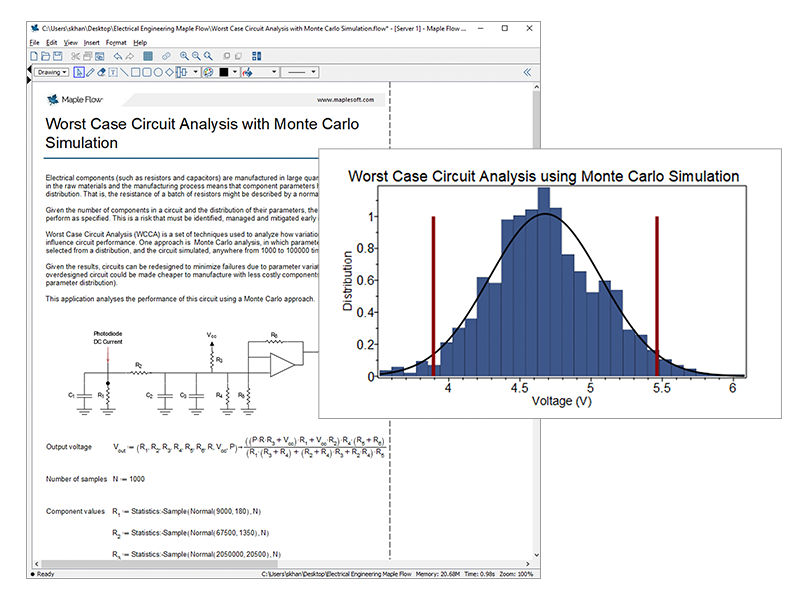Calculation Tool for Electrical Engineers – Maple Flow

# Mathematically-live notebook for Electrical Engineers

From stub matching and circuit analysis to transmission line modeling and semiconductor math, Maple Flow delivers an easy-to-use calculation tool for Electrical EngineersElectrical engineers using Maple Flow to do calculations, make notes, and write design documents in an environment that feels like you’re using paper

Maple Flow provides a freeform, paper-like, calculation environment.

• Do calculations in readable, natural math notation, with full support for units, and easy-to-use solvers
• Create immersive, engaging design reports with text, equations, images and plots
• Use the built-in database of thermophysical properties to extract fluid properties
• Generate attractive plots and charts

# Are You a Mathcad® User?

Special, competitive upgrade pricing is available for Mathcad users switching to Maple Flow.

Do you want to work on mathematically-focused problems, solve larger systems, and develop algorithms? Check out Maple

#FeaturesMathematically Live Paper

Place math, text, plots, and images anywhere on your workspace. Easily move elements into position or reorganize them.Position Determines Evaluation Order

Maple Flow reads and evaluates math from left to right and top to bottom.Automatic Recalculation

Any changes you make to math are automatically cascade throughout the entire document, so results are always up to date.Attractive, Engaging Documents

Maple Flow gives you a clean, uncluttered workspace that allows you to communicate your ideas clearly.Based on the Maple Programming Language

You can use nearly all of Maple’s packages, including a full-featured units system.Easy to Learn, Easy to Use

Maple Flow is the most advanced sheet of “paper” you’ll need to work on calculations. With a low learning curve, you can easily create new documents, debug your work, and lean on a comprehensive set of built-in application examples.What you see in Maple Flow is what you’ll get when you print or share your work as PDF, and you can specify the page size and provide custom headers and footers.

Mathcad is a registered trademark of PTC Inc. or its subsidiaries in the U.S. and in other countries. MATLAB is a registered trademark of The MathWorks, Inc.

## Circuit Analysis Using Transfer Functions and Laplace Transforms

You can use Maple Flow to derive and manipulate transfer functions of electric circuits using Kirchoff’s current and voltage laws. Transfer functions can be converted to differential equations or discretized, and you can easily generate Phase and Magnitude plots.

Transfer functions can contain symbolic coefficients; these parameters can be optimized to match a target response.## Cable Ampacity using the Nehers-McGrath Method

Heat is generated when current flows through a cable. The ampacity of a cable is the amount of current a cable can carry without exceeding its temperature rating. Accurately estimating ampacity is critical to minimizing the total lifetime cost of a cable installation.

This application implements the Nehers-McGrath equations and cross-checks the results against those tabulated in the National Electrical Code (2017); the good agreement means that this worksheet can be the basis of more complex cable ampacity calculations.

This application uses Maple Flow’s built-in units system; units flow from parameter definitions, through equations and into the final results.## Mathematical Modeling of Semiconductor Devices

Semiconductors are complex devices, but Maple Flow helps you derive the math models to accurately describe their characteristics.

MOSFETs are a critical component of modern electronics such as smartphones and other portable devices. Low power MOSFETS are critical to switching in power supply systems.

With Maple Flow, you can turn equivalent circuit models of MOSFETS into analytical equations by writing down and manipulating the basic relationships.## Worst-Case Circuit Analysis

Electrical components are manufactured in large quantities. Inconsistencies in the materials and the manufacturing process mean that component parameters have a statistical distribution. That is, the resistance of a batch of resistors might be described by a normal distribution.

Given the number of components in a circuit and the distribution of their parameters, the circuit may not perform as specified. This is a risk that must be identified, managed and mitigated early in the design process.

Electrical engineers often use Maple Flow for worst-case circuit analysis. You can either employ:

• Monte Carlo analysis, in which parameters are randomly selected from a distribution, and the circuit simulated, anywhere from 1000 to 100000 times. This uses Maple Flow’s tools for
• sampling probability distributions
• element-wise calculations for fast numeric evaluation
• generating histograms and statistical analysis
• or evaluate the circuit equations at the extreme value of all circuit components. This uses Maple Flow’s tools for
• generating permutations of parameters
• element-wise calculations## Stub Matching on a Transmission Line

RF and microwave engineers often need to match load to the impedance of a transmission line. This known as stub matching, and involves numerically solving a nonlinear set of equations.

This requires strong numerical solvers, found in fsolve. This supersedes traditional approaches using Smith charts

The parameters in these problems usually have dimensions (for example, resistances are in ohms and distances are in meters etc).  Maple Flow can carry units from parameter definitions through to the final numerical solution of equations.